# How to keep or remove leading zeros

In the previous post, we talked that how to add leading zeros in the front of a number of text string. And this post explains that how to keep leading zeros as you type in excel. How to prevent automatic truncation of leading zeros in excel. How to remove or delete the leading zeros.

Assuming that you have a list of zip codes in your worksheet, and when you type the zip codes that contains leading zeros, the excel will truncate the leading zeros automatically by default. Such as, you try to type a zip code like “00010086” in a cell, and it will change to “10086”.

If you want to keep the leading zeros as you type in a cell, you need to change the cell format to Text before you type the zip code number. Let’s refer to the following steps:

1# select the range of cells that you want to type the zip codes that contain leading zeros.

2# right click on the selected range, select “Format Cells” from the pop-up menu. The Format Cells window will appear.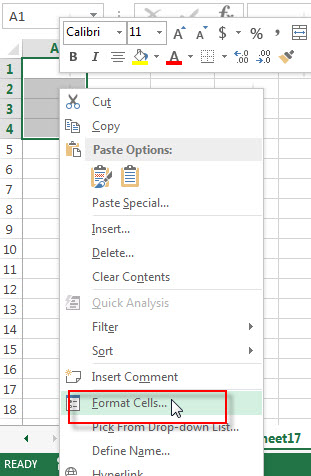3# switch to the Number tab, then choose Text from the Category list.  Click OK button.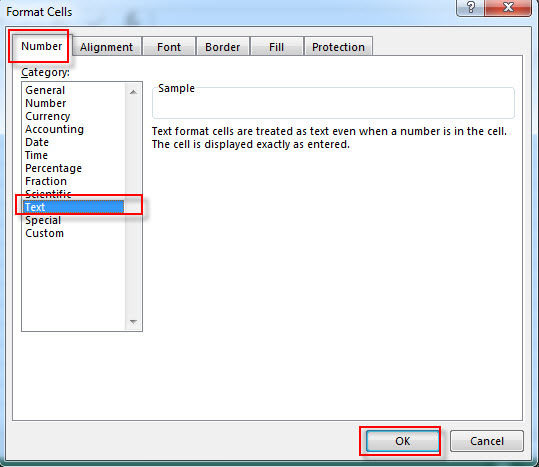4# let’s see the result.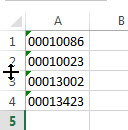Note: you will see a small green triangle in the top-left corner of the cells with leading zeros, it indicates there are some errors with the cell contents. If you want to remove it, you need to select the cells, then click the warning sign, next to click “Ignore Error” from the pop-up menu.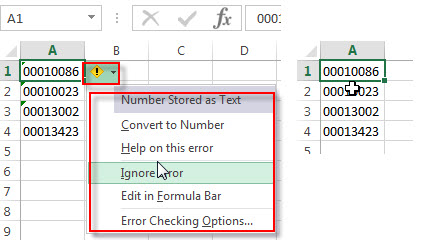There is another way to keep leading zeros as you type, you just need to insert an apostrophe in front of the number you type.  And the apostrophe character will not be displayed in the cell, but excel will treat the contents of the cell as text format.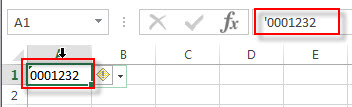If you have a list of phone number with leading zeros, and all numbers are treated as Text format by Excel. if you want to remove all leading zeros in the front of the phone number in a cell, you just need to select the cell,  click exclamation point, then select “Convert to Number” from the pop-up menu.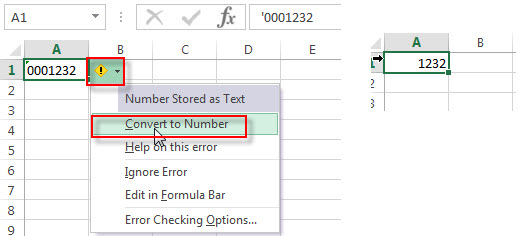Or you can use the VALUE function to convert the text to number.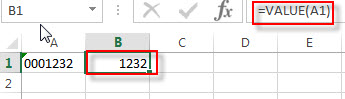## Remove the Leading zeros using VBA macro

You can remove or delete leading zeros in front of the cells with excel VBA macro, just refer to the below steps:

1# click on “Visual Basic” command under DEVELOPER Tab.2# then the “Visual Basic Editor” window will appear.

3# click “Insert” ->”Module” to create a new module4# paste the below VBA code into the code window. Then clicking “Save” button.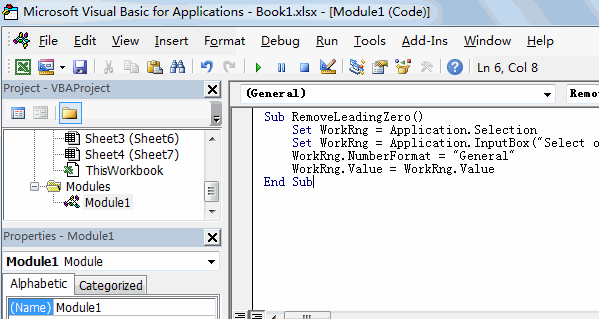```Sub RemoveLeadingZero()
Set WorkRng = Application.Selection
WorkRng.NumberFormat = "General"
WorkRng.Value = WorkRng.Value
End Sub```

5# back to the current worksheet, then run the above excel macro, select the range that you want to delete leading zero.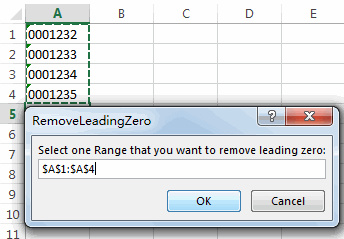6# click “OK” button, you will see that all leading zeros have been removed in the selected range.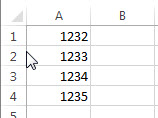### Related Functions

• Excel VALUE function
The Excel VALUE function converts a text value that represents a number to a numeric value.The syntax of the VALUE function is as below:= VALUE  (text)…
• Excel Text function
The Excel TEXT function converts a numeric value into text string with a specified format. The TEXT function is a build-in function in Microsoft Excel and it is categorized as a Text Function. The syntax of the TEXT function is as below: = TEXT (value, Format code)…

### Related Posts

• How to insert leading zeros to number or text
Assuming that you want to insert leading zeros  to number in Cell A2, then you can try to use the TEXT function to create an excel formula. You can also use the CONCATENATE function to add the specific digit of leading zeros into each number…
• Count Numbers with Leading Zeros
Suppost you have a list of product ID in your worksheet, and the product ID contains the different number of leading zeros in the front of string. how to preserve the leading zeros while counting numbers. And you can use another Excel function named as SUMPRODUCT.…
Related Posts

VBA Macro For VLOOKUP From Another Sheet

In the previous post, you should know that how to fix or remove the #N/A error when using VLOOKUP formula to lookup value from another sheet. And this post will show you how to use VBA code to vlookup data ...

How To Insert Comments in Protected Worksheet in Excel

This post will show you how to allow comments in a protected worksheet in Excel. You can easily to insert comments into cells in a normal worksheet in Excel, but if want to insert a comment in a worksheet that ...

How To Convert Text to Upper Cases(Using VBA) in Excel

This post will show you how to switch from lower case to upper case in Excel. and I am going to show you two different ways of converting text to upper cases using formula or VBA macro in Excel 2013,Excel ...

How To Hide Every Other Row in Excel (Using VBA)

This post will show you how to hide alternate rows or columns in Excel or how to hide every third, fourth, fifth row or column in Excel. If you want to hide every other row in your current worksheet, how ...

How to Disable the Save As Prompt in Excel

This post will show you how to use a VBA Macro to save an Excel file and overwrite any existing file without a prompt so that you are going to get the little window that says file already exists do ...

How to Count Cells that Contain even or odd numbers in Excel

This post will guide you how to count the number of cells that contain odd or even numbers within a range of cells using a formula in Excel 2013/2016.How do I count cells that contain odd numbers through the use ...

How to Count Cells that Contain negative Numbers in Excel

This post will guide you how to count the number of cells that contain negative numbers within a range of cells using a formula in Excel 2013/2016.You can count the number of negative numbers in your data using easy functions ...

How to Count Cells Are Not Blank or Empty in Excel

This post will guide you how to count cells that are not blank or empty in a given range cells using a formula in Excel 2013/2016.How do I count the number of cells that are not blank in a particular ...

How to Count Cells Not equal to X or Y in Excel

This post will guide you how to count the number of cells not equal to criteria X or Y in a given range cells using a formula in Excel 2013/2016.You can easily to count cells equal to or not equal ...

How to Count Cells Less Than a Specific Value in Excel

This post will guide you how to count the number of cells less than a particular numeric value in a given range cells using a formula in Excel 2013/2016. How do I count cells that are less than a specific ...

Sidebar